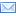Email this page## Alternative Computational Algorithms, Grades 3 – 6

Using base ten blocks, participants will find that we don’t have to start on the right side when we add and subtract, that we don’t have to borrow in subtraction EVER if we don’t want to, that we can multiply 2-digit numbers in our heads, and that we can divide however we want to, so that not everyone’s written solution looks the same. We will also see that we can FOIL fraction multiplication, instead of changing mixed numbers into improper fractions, and that we can do division by partial quotients in fractions instead of inverting and multiplying.